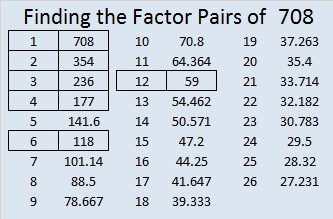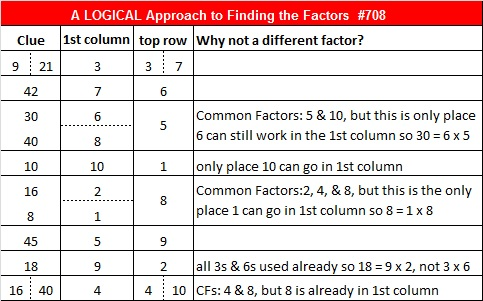# 708 and Level 5

• 708 is a composite number.
• Prime factorization: 708 = 2 x 2 x 3 x 59, which can be written 708 = (2^2) x 3 x 59
• The exponents in the prime factorization are 2, 1, and 1. Adding one to each and multiplying we get (2 + 1)(1 + 1)(1 + 1) = 3 x 2 x 2 = 12. Therefore 708 has exactly 12 factors.
• Factors of 708: 1, 2, 3, 4, 6, 12, 59, 118, 177, 236, 354, 708
• Factor pairs: 708 = 1 x 708, 2 x 354, 3 x 236, 4 x 177, 6 x 118, or 12 x 59
• Taking the factor pair with the largest square number factor, we get √708 = (√4)(√177) = 2√177 ≈ 26.608269.Here is a factor tree for 708:Today’s factoring puzzle reminds me of lumps of coal:Print the puzzles or type the solution on this excel file: 10 Factors 2015-12-07

———————————————————————————

Here are a few more facts about 708:

708 can be written as the sum of consecutive numbers three different ways:

• 235 + 236 + 237 = 708; that’s 3 consecutive numbers.
• 85 + 86 + 87 + 88 + 89 + 90 + 91 + 92 = 708; that’s 8 consecutive numbers.
• 18 + 19 + 20 + 21 + 22 + 23 + 24 + 25 + 26 + 27 + 28 + 29 + 30 + 31 + 32 + 33 + 34 + 35 + 36 + 37 + 38 + 39 + 40 + 41 = 708; that’s 24 consecutive numbers.

708 is palindrome 323 in BASE 15; note that 3(225) + 2(15) + 3(1) = 708.

———————————————————————————This site uses Akismet to reduce spam. Learn how your comment data is processed.